# A look on income increment and poverty in SingaporeQuek Zhan Hong Sheriff

Sheriff -DS102 Project

# Name: SheriffDS102 | Course Assignment

Introduction

This report aims to investigate the trends of income across deciles in Singapore.

• What are the changes in income?

• Is income increment consistent across deciles
• Is income increasing because of adjustments to inflation or are people actually getting paid better
• Is income enough to afford cost of living in Singapore?

• Income inequality is existent but has Singapore helped minimise income inequality gap?
• Is Singapore becoming unaffordable or is consumerism increasing
• How does Singapore fare against other countries' poverty metrics

Dataset
Dataset for average household income and expenditure was obtained from Department of Statistics Singapore
Dataset for consumer price index was obtained from Data.gov.sg

Description
Several data were obtained from Department of Statistics:

• Average Monthly Household income (Excluding Employer CPF contribution) from 2000 to 2017
• Average Household Expenditure from 2000 to 2017

Data for Household income provides the monthly income of the nation per year and the average monthly income per decile (0-10%, 11-20%, 21-30%, 31-40%, 41-50%, 51-60%, 61-70%, 71-80%, 81-90%, 91-100%) where 0-10% is the lowest income group. Data provided is based on nominal income.

Data for Household expenditure provides national average nominal expenditure and average for deciles per 20% of the population. Changes in expenditure adjusted to inflation (real expenditure) will allow us to investigate if expenditure has increased or solely adjusted to inflation rates.

Consumer price index (CPI) was obtained from data.gov which provides the comprehensive breakdown of the CPI per category per year from 1976-2017. Each main category eg. Food, provides the comprehensive breakdown of expenditure across each income group. The average for CPI per year would give an estimation of the chages in inflation rate from 2000-2017 to measure the real change in income over this duration.

In :
import pandas as pd
import numpy as np
import matplotlib.pyplot as plt
import seaborn as sns
sns.set()
pd.options.display.float_format = '{:.2f}'.format
pd.options.mode.chained_assignment = None


Data Cleaning

Initial reading of monthly household income shows that the data is presented as strings, hence we will need to convert the entire dataframe into integers.

In :
avg_inc = pd.read_csv('Average Monthly Household Income from Work (Excluding Employer CPF Contributions) Among Resident Employed Households by Deciles, 2000 - 2017.csv',
skiprows = 3, nrows = 12).dropna(axis =1)

# Transpose the dataframe for easier viewing
avg_inc = avg_inc.T
# Remove white spaces in the dataset
avg_inc.columns = avg_inc.columns.str.strip()

# Creating an index of the same dtype as Consumer price index dataframe
# To ensure common index, we used an integer index of years
avg_inc.Deciles = avg_inc.Deciles.apply(int)
avg_inc.set_index(avg_inc.Deciles, inplace = True)

#Removing the extra column of years
avg_inc.drop(columns = ['Deciles'], inplace = True)

#Removing the comma in each value in the cell
avg_inc = avg_inc.applymap(lambda x:x.replace(',',''))

#Converting all strings into integers
avg_inc = avg_inc.apply(pd.to_numeric)

avg_inc

Out:
Total 1st - 10th 11th - 20th 21st - 30th 31st - 40th 41st - 50th 51st - 60th 61st - 70th 71st - 80th 81st - 90th 91st - 100th
Deciles
2000 5456 1285 2062 2737 3367 4097 4830 5773 6919 8631 14862
2001 5736 1209 2040 2717 3434 4149 5015 5971 7365 9557 15905
2002 5572 1151 1956 2627 3312 4043 4884 5891 7187 9144 15524
2003 5618 1112 1942 2668 3330 4103 4981 5936 7273 9142 15688
2004 5761 1140 2009 2721 3431 4200 4978 6005 7256 9443 16425
2005 6052 1162 2064 2833 3645 4390 5301 6458 7846 9797 17021
2006 6280 1165 2114 2903 3673 4514 5477 6535 8046 10203 18170
2007 6889 1223 2218 3078 3950 4870 5962 7234 8694 11491 20174
2008 7752 1300 2464 3464 4420 5455 6753 8107 9849 12916 22797
2009 7549 1264 2462 3436 4495 5391 6601 7972 9733 12354 21784
2010 8058 1385 2679 3759 4887 5959 7090 8450 10142 12887 23345
2011 8864 1460 2834 3988 5200 6303 7587 9147 11193 14307 26622
2012 9515 1518 2985 4290 5529 6800 8196 9806 11973 15038 29012
2013 9597 1574 3045 4467 5696 7135 8479 10159 12521 15609 27287
2014 10244 1621 3273 4676 6144 7423 9082 10693 13188 16601 29742
2015 10515 1745 3402 4965 6262 7746 9480 11323 13519 16848 29860
2016 10493 1732 3486 5040 6434 7856 9636 11421 13765 17188 28377
2017 10920 1763 3512 5158 6554 8254 9736 12042 14351 17809 30018

Now that we have cleaned the nominal household income dataset, we will need to deduct personal CPF and income tax before we can convert into real income. Based on the CPF metrics, we made an assumption that all income providers are aged 55 and below since we do not have access to income and demographics. Hence according to CPF, we factor in a 17% deduction with \$8000 income cap.

Another key information we are missing was the number of average working adults per household in each income decile. The average household size from 2000-2017 range from 3.3-3.7, and hence we made the assumption that each household has 2 working adults and 1-2 non-working family member. This is to account for overestimation of deductions made to the nominal income.

In :
#CPF deduction

# Saved the original copy of the nominal income without deductions
ori_inc = avg_inc.copy()

#Reset the index for plotting
ori_inc.reset_index(inplace = True)

Out:
Deciles Total 1st - 10th 11th - 20th 21st - 30th 31st - 40th 41st - 50th 51st - 60th 61st - 70th 71st - 80th 81st - 90th 91st - 100th
0 2000 5456 1285 2062 2737 3367 4097 4830 5773 6919 8631 14862
1 2001 5736 1209 2040 2717 3434 4149 5015 5971 7365 9557 15905
2 2002 5572 1151 1956 2627 3312 4043 4884 5891 7187 9144 15524
3 2003 5618 1112 1942 2668 3330 4103 4981 5936 7273 9142 15688
4 2004 5761 1140 2009 2721 3431 4200 4978 6005 7256 9443 16425
In :
# Deducting CPF contribution for each cell

# Creating a function to deduct CPF
def cpf(x):
per_income = x/2
if per_income <= 8000:
return (per_income*0.83)*2
else:
return (x -(8000*0.17*2))

avg_inc = avg_inc.applymap(lambda x: cpf(x))

# Nominal income with CPF deductions
avg_inc

Out:
Total 1st - 10th 11th - 20th 21st - 30th 31st - 40th 41st - 50th 51st - 60th 61st - 70th 71st - 80th 81st - 90th 91st - 100th
Deciles
2000 4528.48 1066.55 1711.46 2271.71 2794.61 3400.51 4008.90 4791.59 5742.77 7163.73 12335.46
2001 4760.88 1003.47 1693.20 2255.11 2850.22 3443.67 4162.45 4955.93 6112.95 7932.31 13201.15
2002 4624.76 955.33 1623.48 2180.41 2748.96 3355.69 4053.72 4889.53 5965.21 7589.52 12884.92
2003 4662.94 922.96 1611.86 2214.44 2763.90 3405.49 4134.23 4926.88 6036.59 7587.86 13021.04
2004 4781.63 946.20 1667.47 2258.43 2847.73 3486.00 4131.74 4984.15 6022.48 7837.69 13705.00
2005 5023.16 964.46 1713.12 2351.39 3025.35 3643.70 4399.83 5360.14 6512.18 8131.51 14301.00
2006 5212.40 966.95 1754.62 2409.49 3048.59 3746.62 4545.91 5424.05 6678.18 8468.49 15450.00
2007 5717.87 1015.09 1840.94 2554.74 3278.50 4042.10 4948.46 6004.22 7216.02 9537.53 17454.00
2008 6434.16 1079.00 2045.12 2875.12 3668.60 4527.65 5604.99 6728.81 8174.67 10720.28 20077.00
2009 6265.67 1049.12 2043.46 2851.88 3730.85 4474.53 5478.83 6616.76 8078.39 10253.82 19064.00
2010 6688.14 1149.55 2223.57 3119.97 4056.21 4945.97 5884.70 7013.50 8417.86 10696.21 20625.00
2011 7357.12 1211.80 2352.22 3310.04 4316.00 5231.49 6297.21 7592.01 9290.19 11874.81 23902.00
2012 7897.45 1259.94 2477.55 3560.70 4589.07 5644.00 6802.68 8138.98 9937.59 12481.54 26292.00
2013 7965.51 1306.42 2527.35 3707.61 4727.68 5922.05 7037.57 8431.97 10392.43 12955.47 24567.00
2014 8502.52 1345.43 2716.59 3881.08 5099.52 6161.09 7538.06 8875.19 10946.04 13881.00 27022.00
2015 8727.45 1448.35 2823.66 4120.95 5197.46 6429.18 7868.40 9398.09 11220.77 14128.00 27140.00
2016 8709.19 1437.56 2893.38 4183.20 5340.22 6520.48 7997.88 9479.43 11424.95 14468.00 25657.00
2017 9063.60 1463.29 2914.96 4281.14 5439.82 6850.82 8080.88 9994.86 11911.33 15089.00 27298.00

Income taxes is chargable annually after cpf contribution. In this deduction, we converted the household income by annual and did the respective tax deductions by tax brackets. We also assumed no financial relief or grants computed into accessible income.
Respective income tax rates were obtained from data.gov.sg with data from 2003 to 2017. Since we do not have tax rate from 2000 to 2002, we will assume the same tax rate as of 2003.

In :
# Income tax deduction based on Singapore IRAS metrics

# Creating a function to deduct income by tax brackets for each respective years, again assuming 2 working adults

#Tax rate from 2000 to 2005
def tax_deduct0005(x):
b = x/2
a = b*12
if a <= 20000:
return x
elif a <=30000:
return ((a-((a-20000)*0.04))/12.0)*2
elif a <= 40000:
return ((a-(((a-30000)*0.06)+200))/12.0)*2
elif a <= 80000:
return ((a-(((a-40000)*0.09)+550))/12.0)*2
elif a <= 160000:
return ((a-(((a-120000)*0.15)+7950))/12.0)*2
elif a <= 320000:
return ((a-(((a-200000)*0.19)+20750))/12.0)*2
else:
return ((a-(((a-320000)*0.22)+42350))/12.0)*2

#Tax rate for 2006
def tax_deduct06(x):
b = x/2
a = b*12
if a <= 20000:
return x
elif a <=30000:
return ((a-((a-20000)*0.0375))/12.0)*2
elif a <= 40000:
return ((a-(((a-30000)*0.0575)+200))/12.0)*2
elif a <= 80000:
return ((a-(((a-40000)*0.0875)+550))/12.0)*2
elif a <= 160000:
return ((a-(((a-120000)*0.145)+7950))/12.0)*2
elif a <= 320000:
return ((a-(((a-200000)*0.18)+20750))/12.0)*2
else:
return ((a-(((a-320000)*0.21)+42350))/12.0)*2

#Tax rate from 2007 -2011
def tax_deduct0711(x):
b = x/2
a = b*12
if a <= 20000:
return x
elif a <=30000:
return ((a-((a-20000)*0.035))/12.0)*2
elif a <= 40000:
return ((a-(((a-30000)*0.055)+200))/12.0)*2
elif a <= 80000:
return ((a-(((a-40000)*0.085)+550))/12.0)*2
elif a <= 160000:
return ((a-(((a-120000)*0.14)+7950))/12.0)*2
elif a <= 320000:
return ((a-(((a-200000)*0.17)+20750))/12.0)*2
else:
return ((a-(((a-320000)*0.20)+42350))/12.0)*2

#Tax rate from 2012-2016
def tax_deduct1216(x):
b = x/2
a = b*12
if a <= 20000:
return x
elif a <=30000:
return ((a-((a-20000)*0.02))/12.0)*2
elif a <= 40000:
return ((a-(((a-30000)*0.035)+200))/12.0)*2
elif a <= 80000:
return ((a-(((a-40000)*0.07)+550))/12.0)*2
elif a <= 120000:
return ((a-(((a-30000)*0.115)+3350))/12.0)*2
elif a <= 160000:
return ((a-(((a-120000)*0.15)+7950))/12.0)*2
elif a <= 200000:
return ((a-(((a-160000)*0.17)+13950))/12.0)*2
elif a <= 320000:
return ((a-(((a-200000)*0.18)+20750))/12.0)*2
else:
return ((a-(((a-320000)*0.20)+42350))/12.0)*2

#Tax rate from 2017 onwards
def tax_deduct17(x):
b = x/2
a = b*12
if a <= 20000:
return x
elif a <=30000:
return ((a-((a-20000)*0.02))/12.0)*2
elif a <= 40000:
return ((a-(((a-30000)*0.035)+200))/12.0)*2
elif a <= 80000:
return ((a-(((a-40000)*0.07)+550))/12.0)*2
elif a <= 120000:
return ((a-(((a-30000)*0.115)+3350))/12.0)*2
elif a <= 160000:
return ((a-(((a-120000)*0.15)+7950))/12.0)*2
elif a <= 200000:
return ((a-(((a-160000)*0.18)+13950))/12.0)*2
elif a <= 320000:
return ((a-(((a-200000)*0.19)+20750))/12.0)*2
else:
return ((a-(((a-320000)*0.22)+42350))/12.0)*2

# Different tax rates are calculated for each respective year
# First we transpose the dataset so that we can apply the function by year

avg_inc = avg_inc.T

for i in avg_inc:
if int(i) <= 2005:
avg_inc[i] = avg_inc[i].apply(lambda x: tax_deduct0005(x))
elif int(i) == 2006:
avg_inc[i] = avg_inc[i].apply(lambda x: tax_deduct06(x))
elif int(i) <= 2011:
avg_inc[i] = avg_inc[i].apply(lambda x: tax_deduct0711(x))
elif int(i) <= 2016:
avg_inc[i] = avg_inc[i].apply(lambda x: tax_deduct1216(x))
else:
avg_inc[i] = avg_inc[i].apply(lambda x: tax_deduct17(x))

#Transpose the dataframe back
avg_inc = avg_inc.T

#Calculate new total average income after deductions
for i in range(18):
avg_inc['Total'].iloc[i]= (avg_inc.iloc[i,1:].mean())

#Nominal income with deductions
avg_inc

Out:
Total 1st - 10th 11th - 20th 21st - 30th 31st - 40th 41st - 50th 51st - 60th 61st - 70th 71st - 80th 81st - 90th 91st - 100th
Deciles
2000 4438.31 1066.55 1711.46 2271.71 2794.61 3397.82 3981.88 4733.26 5664.87 7027.33 11733.60
2001 4652.25 1003.47 1693.20 2255.11 2850.22 3439.26 4129.29 4891.03 6012.84 7726.74 12521.38
2002 4523.75 955.33 1623.48 2180.41 2748.96 3354.80 4024.90 4827.28 5873.96 7414.80 12233.61
2003 4559.29 922.96 1611.86 2214.44 2763.90 3402.60 4102.19 4863.14 5941.06 7413.29 12357.48
2004 4711.03 946.20 1667.47 2258.43 2847.73 3479.89 4099.80 4918.12 5927.80 7640.63 13324.25
2005 4947.49 964.46 1713.12 2351.39 3025.35 3631.29 4357.17 5305.20 6388.12 7908.01 13830.85
2006 5136.70 966.95 1754.62 2409.49 3048.59 3731.12 4500.44 5366.33 6585.51 8219.16 14784.75
2007 5627.91 1015.09 1840.94 2554.74 3278.50 4017.29 4891.93 5915.65 7077.66 9201.84 16485.44
2008 6329.23 1079.00 2045.12 2875.12 3656.87 4485.85 5538.38 6631.86 7954.82 10284.06 18741.22
2009 6160.37 1049.12 2043.46 2851.88 3716.94 4434.59 5419.16 6494.50 7866.73 9857.25 17870.04
2010 6576.05 1149.55 2223.57 3119.97 4030.91 4889.53 5802.71 6892.35 8177.34 10262.03 19212.50
2011 7230.20 1211.80 2352.22 3310.04 4281.61 5185.42 6192.53 7421.69 8975.52 11340.45 22030.72
2012 7771.45 1259.94 2477.55 3556.15 4563.96 5588.13 6701.49 7944.25 9616.96 11982.83 24023.20
2013 7824.73 1306.42 2527.35 3700.12 4699.79 5856.44 6919.94 8216.73 10039.96 12423.59 22556.95
2014 8258.91 1345.43 2716.59 3870.13 5062.70 6087.12 7385.40 8628.93 10554.82 12301.35 24636.59
2015 8475.29 1448.35 2823.66 4105.20 5157.22 6345.83 7692.61 9115.22 10810.32 12519.95 24734.53
2016 8453.44 1437.56 2893.38 4166.20 5294.98 6433.93 7813.03 9190.87 11000.20 12820.85 23483.45
2017 8802.06 1463.29 2914.96 4262.18 5391.09 6746.26 7890.22 9670.22 11452.54 13370.43 24859.36

Next, we draw data for the consumer price index. Since we have a comprehensive breakdown per year, we take the average to find the CPI per year.

In :
#Creating df for consumer price index

#Filter Data for year 2000 and above
cpi = cpi[cpi.year > 1999]

Out:
year level_1 level_2 value
468 2000 All Items Food 73.956
469 2000 All Items Food Excl Food Servicing Services 70.173
470 2000 All Items Food Servicing Services 76.754
471 2000 All Items Clothing & Footwear 93.094
472 2000 All Items Housing & Utilities 65.886
473 2000 All Items Household Durables And Services 87.145
474 2000 All Items Health Care 66.768
475 2000 All Items Transport 76.125
476 2000 All Items Communication 107.936
477 2000 All Items Recreation & Culture 88.91
478 2000 All Items Education 63.633
479 2000 All Items Miscellaneous Goods & Services 73.188
480 2001 All Items Food 74.324
481 2001 All Items Food Excl Food Servicing Services 69.881
482 2001 All Items Food Servicing Services 77.687
In :
#Modify the df to display average CPI per year
cpi = cpi[['year','value']]
cpi.value = cpi.value.astype('float')
cpi = cpi.groupby('year').mean()

# Reset the index to match with the income dataframe to calculate real income
cpi.reset_index(inplace= True)
cpi.set_index('year', inplace = True)
cpi

Out:
value
year
2000 78.63
2001 79.30
2002 79.34
2003 80.04
2004 81.55
2005 82.03
2006 82.73
2007 84.48
2008 88.58
2009 89.29
2010 90.83
2011 93.70
2012 96.51
2013 98.56
2014 99.98
2015 100.30
2016 100.90
2017 101.98
In :
#Creating a new df by dividing each income with their respective CPI to obtain real income based on 2017
avg_inc = avg_inc.T

#Real income with deductions
real_inc = avg_inc.div(cpi.iloc[:,0], axis = 'columns')

#CPI for 2017 is 101.976750
real_inc = real_inc.apply(lambda x: x*101.976750)

#Transpose the df for plotting
real_inc = real_inc.T

#Real income with deductions

Out:
Total 1st - 10th 11th - 20th 21st - 30th 31st - 40th 41st - 50th 51st - 60th 61st - 70th 71st - 80th 81st - 90th 91st - 100th
Deciles
2000 5756.08 1383.22 2219.61 2946.20 3624.35 4406.66 5164.13 6138.60 7346.82 9113.80 15217.40
2001 5982.45 1290.39 2177.33 2899.90 3665.17 4422.63 5309.95 6289.49 7732.06 9936.00 16101.56
2002 5814.10 1227.83 2086.56 2802.34 3533.07 4311.71 5172.96 6204.20 7549.44 9529.78 15723.10
2003 5808.60 1175.86 2053.53 2821.22 3521.24 4334.96 5226.25 6195.70 7568.99 9444.62 15743.59
2004 5890.88 1183.17 2085.08 2824.04 3560.93 4351.41 5126.58 6149.83 7412.38 9554.18 16661.23
In :
#Reset index for plotting of nominal income with deductions
avg_inc = avg_inc.T
avg_inc.reset_index(inplace=True)

Out:
Deciles Total 1st - 10th 11th - 20th 21st - 30th 31st - 40th 41st - 50th 51st - 60th 61st - 70th 71st - 80th 81st - 90th 91st - 100th
0 2000 4438.31 1066.55 1711.46 2271.71 2794.61 3397.82 3981.88 4733.26 5664.87 7027.33 11733.60
1 2001 4652.25 1003.47 1693.20 2255.11 2850.22 3439.26 4129.29 4891.03 6012.84 7726.74 12521.38
2 2002 4523.75 955.33 1623.48 2180.41 2748.96 3354.80 4024.90 4827.28 5873.96 7414.80 12233.61
3 2003 4559.29 922.96 1611.86 2214.44 2763.90 3402.60 4102.19 4863.14 5941.06 7413.29 12357.48
4 2004 4711.03 946.20 1667.47 2258.43 2847.73 3479.89 4099.80 4918.12 5927.80 7640.63 13324.25
In :
#Reset index for plotting of real income
real_inc.reset_index(inplace = True)
real_inc.rename(columns = {'Deciles': 'Year'}, inplace= True)

Out:
Year Total 1st - 10th 11th - 20th 21st - 30th 31st - 40th 41st - 50th 51st - 60th 61st - 70th 71st - 80th 81st - 90th 91st - 100th
0 2000 5756.08 1383.22 2219.61 2946.20 3624.35 4406.66 5164.13 6138.60 7346.82 9113.80 15217.40
1 2001 5982.45 1290.39 2177.33 2899.90 3665.17 4422.63 5309.95 6289.49 7732.06 9936.00 16101.56
2 2002 5814.10 1227.83 2086.56 2802.34 3533.07 4311.71 5172.96 6204.20 7549.44 9529.78 15723.10
3 2003 5808.60 1175.86 2053.53 2821.22 3521.24 4334.96 5226.25 6195.70 7568.99 9444.62 15743.59
4 2004 5890.88 1183.17 2085.08 2824.04 3560.93 4351.41 5126.58 6149.83 7412.38 9554.18 16661.23

In the first plot, we look at how income deductions and conversion into real income affects the income trend. This allows us to evaluate which model will be better for comparison.

In :
# We used 3 different income deciles to look at the overall trend across decile groups
# Here we chose the 1-10th, 51-60th and 91-100th groups to ensure a throughout evaluation
fig = plt.figure(figsize = (15,20))
ori_inc.plot(x = 'Deciles', y = '1st - 10th', ax = ax0)
avg_inc.plot(x = 'Deciles', y = '1st - 10th', ax = ax0)
real_inc.plot(x = 'Year', y = '1st - 10th', ax = ax0)
ax0.set_xticks(np.arange(2000, 2018, 1))
ax0.set_ylabel('SGD')
ax0.set_title("Comparison of nominal and real income with and without CPF/tax deductions for 1st - 10th decile")
ax0.legend(['Nominal income without deductions', 'Nominal income with deductions', 'Real income with deductions'])

ori_inc.plot(x = 'Deciles', y = '51st - 60th', ax = ax1)
avg_inc.plot(x = 'Deciles', y = '51st - 60th', ax = ax1)
real_inc.plot(x = 'Year', y = '51st - 60th', ax = ax1)
ax1.set_xticks(np.arange(2000, 2018, 1))
ax1.set_ylabel('SGD')
ax1.set_title("Comparison of nominal and real income with and without CPF/tax deductions for 51st - 60th decile")
ax1.legend(['Nominal income without deductions', 'Nominal income with deductions', 'Real income with deductions'])

ori_inc.plot(x = 'Deciles', y = '91st - 100th', ax = ax2)
avg_inc.plot(x = 'Deciles', y = '91st - 100th', ax = ax2)
real_inc.plot(x = 'Year', y = '91st - 100th', ax = ax2)
ax2.set_xticks(np.arange(2000, 2018, 1))
ax2.set_ylabel('SGD')
ax2.set_title("Comparison of nominal and real income with and without CPF/tax deductions for 91st - 100th decile")
ax2.legend(['Nominal income without deductions', 'Nominal income with deductions', 'Real income with deductions'])

plt.show()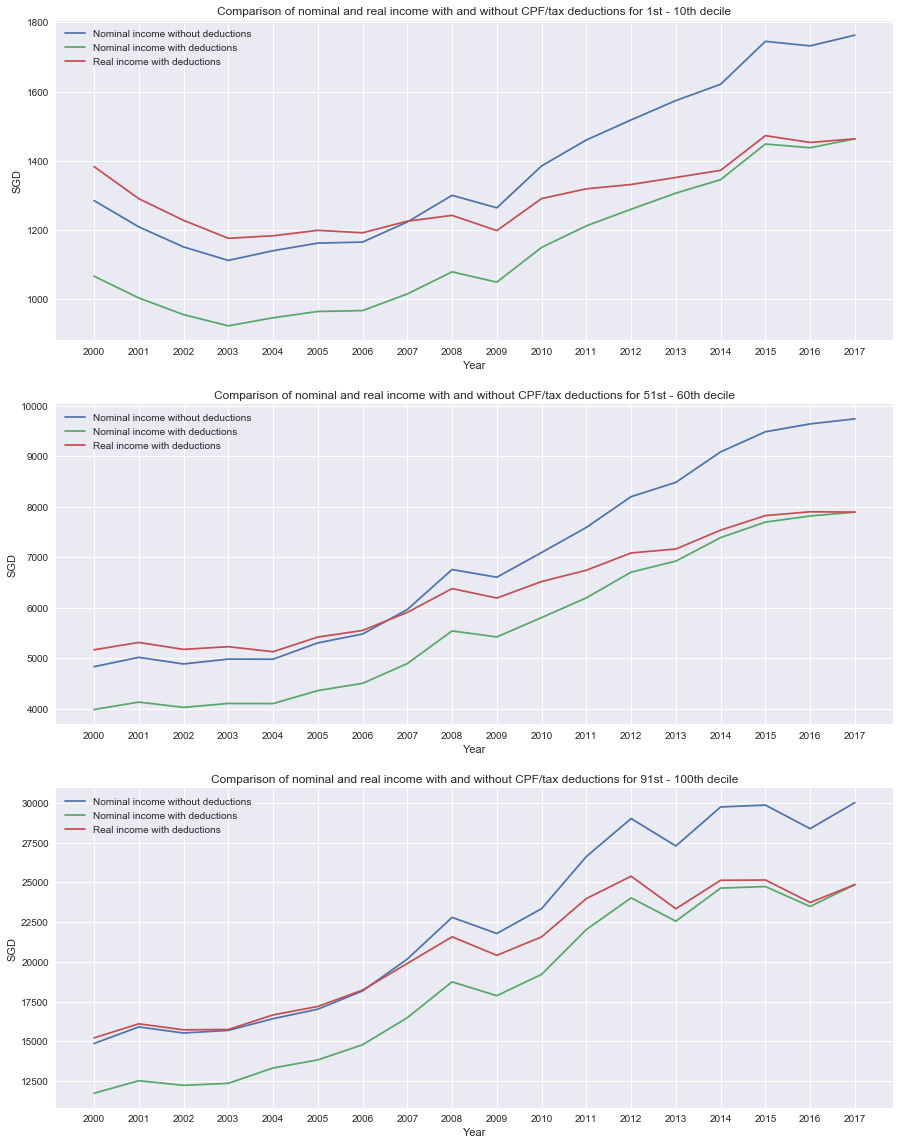First, we account for CPF and tax deduction in accordance to Singapore Standards For CPF we gave the assumption the general working population is aged 54 and below hence 17% CPF deduction was computed. After that we converted nominal income into real income based on Consumer Price Index from each year. We assumed that no financial relief or donations were made in the average income
Comparing the plots in different decile groups, the effect of converting nominal to real income and accounting for tax deductions significantly affect the trend in income changes across all groups. While the shape of the trend remain similar, the extend of increase in income variates significantly. This thus justify the use of real income with deductions for subsequent plotting.

In the next plot, we look at the income trend for real income across decile groups to identify trends in income increment

In :
#Plotting real income across all deciles
from matplotlib import cm
fig = plt.figure(figsize = (15,10))
ax = fig.subplots()
cmap = cm.get_cmap('Spectral')
real_inc.plot(kind = 'line',x ='Year' ,ax= ax, cmap = cmap)

ax.set_xticks(np.arange(2000, 2018))
ax.set_xlabel('Year')
ax.set_title('Changes in Real Disposable Income from 2000-2017 based on 2017 Consumer Price Index')
ax.set_ylabel("Real Income")
ax.set_yticks(np.arange(0, 25000,1000))

plt.show()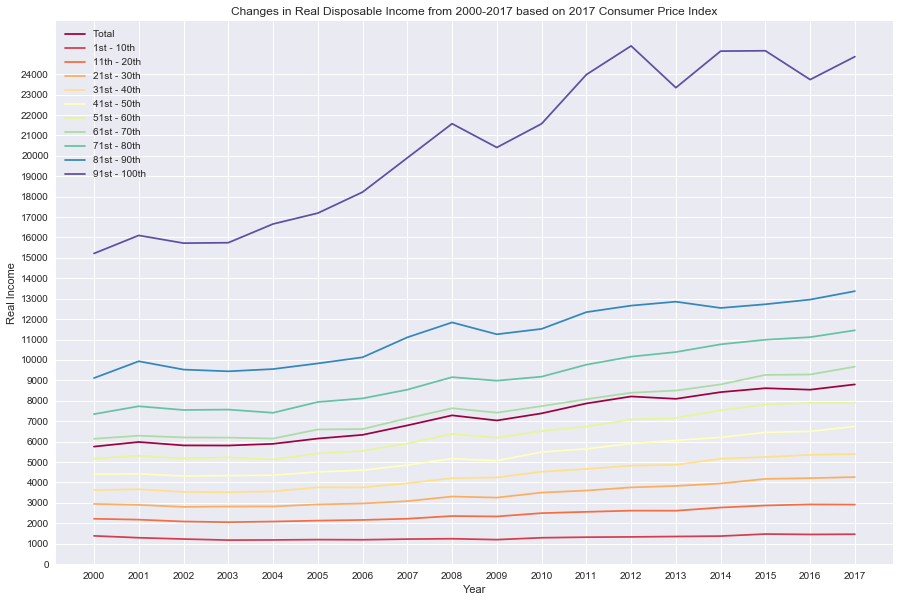As we can see from the plot, there is definite income inequality between the high and low deciles. However, the widening gap across income deciles are mild, except for 91-100th decile group. Furthermore, there is some income increment across deciles, althought the degree increases as we progress up each group.
However, it is alarming to see that income increment is minimal in low decile groups of 10th and below, meaning increment served to adjust to inflation rates. However, further investigate must be taken to determine if the income is sufficient for living in Singapore.

From the plot, the trend suggest that the national average income is similar in trend to the 61-70th decile of the population. Based on that we can see that about 60% of incomes fall below the national average. However, this alone cannot ascertain whether Singaporeans are able to support the cost of living or not. We will need to use other metrics of poverty measurement and also investiate expenditure of different deciles.

To proceed with the next part of the project, we obtained the expenditure report for Singapore. Since one report was conduxted every 5 years, we had to read each data individually, then merge the data into one dataframe.

In :
#Obtain df for expenditure in Singapore across income deciles
#expenditure was only measured in 2002/2003, 2007/2008 and 2012/2013 hence we will need to combine the data and conduct some extrapolation
exp0203 = pd.read_csv('Average Monthly Household Expenditure by Types of Goods and Services (Detailed) and Income Quintile1: 2002 : 03.csv', skiprows = 5, nrows = 345)
exp0708 = pd.read_csv('Average Monthly Household Expenditure by Types of Goods and Services (Detailed) and Income Quintile 1: 2007 : 08.csv', skiprows = 5, nrows = 345)
exp1213 = pd.read_csv('Average Monthly Household Expenditure by Type of Goods and Services (Detailed) and Income Quintile 1: 2012 : 13.csv', skiprows = 5, nrows = 345)

In :
#remove NaN columns for all df in expenditure
exp0203.columns = exp0203.columns.str.strip()
exp0203.drop('Unnamed: 7', axis =1, inplace = True)
exp0708.columns = exp0708.columns.str.strip()
exp0708.drop('Unnamed: 7', axis =1, inplace = True)
exp1213.columns = exp1213.columns.str.strip()
exp1213.drop('Unnamed: 7', axis =1, inplace =True)

In :
#Each dataframe shows the average expenditure and specific breakdown
#However, we only require the average expenditure for this study

Out:
.1 1st - 20th 21st - 40th 41st - 60th 61st - 80th 81st - 100th
0 TOTAL 3,351.9 1,704.2 2,459.7 3,177.5 4,066.8 5,351.4
1 FOOD AND NON-ALCOHOLIC BEVERAGES 332.2 266.8 331.7 342.6 369.3 350.7
2 FOOD 311.9 250.4 311.7 321.1 347.2 329.2
3 Bread and Cereals 60.4 47.2 60.2 61 69.6 63.9
4 Rice 11.4 12.5 14.2 12 10.6 7.9
In :
#Select for expenditure excluding rentals for owner-occupiers since these expenditure is not considered essential for daily living
#exp.head(1) gives us the total expenditure without rentals

#Combining all three dataframe into one dataframe
com_exp = pd.concat([exp1,exp2, exp3])
com_exp.drop(columns = [''], inplace = True)
com_exp = com_exp.applymap(lambda x: x.replace(',',''))
com_exp = com_exp.apply(pd.to_numeric)
com_exp

Out:
.1 1st - 20th 21st - 40th 41st - 60th 61st - 80th 81st - 100th
0 3351.90 1704.20 2459.70 3177.50 4066.80 5351.40
0 3809.10 1786.70 2950.30 3601.60 4569.10 6137.50
0 4724.50 2230.70 3535.50 4698.70 5589.80 7567.80

We realise that the report was conducted from October the previous year till September. Since, most of the expenditure consist of the latest year, we assumed the data to follow the latest year.

In :
#Tidying up the df
com_exp = com_exp.rename(columns = {'.1': 'Total'})

#Data was collected from oct in the previous year to sept in the following year sowe will assume data as the latest year recorded.
com_exp.set_index([[2003,2008,2013]], inplace= True)

# In order to calculate with CPI, we need to expand the dataframe to include missing years
year = np.arange(2000,2018,1)
com_exp = com_exp.reindex(year)
com_exp = com_exp.T
com_exp

Out:
2000 2001 2002 2003 2004 2005 2006 2007 2008 2009 2010 2011 2012 2013 2014 2015 2016 2017
Total nan nan nan 3351.90 nan nan nan nan 3809.10 nan nan nan nan 4724.50 nan nan nan nan
1st - 20th nan nan nan 1704.20 nan nan nan nan 1786.70 nan nan nan nan 2230.70 nan nan nan nan
21st - 40th nan nan nan 2459.70 nan nan nan nan 2950.30 nan nan nan nan 3535.50 nan nan nan nan
41st - 60th nan nan nan 3177.50 nan nan nan nan 3601.60 nan nan nan nan 4698.70 nan nan nan nan
61st - 80th nan nan nan 4066.80 nan nan nan nan 4569.10 nan nan nan nan 5589.80 nan nan nan nan
81st - 100th nan nan nan 5351.40 nan nan nan nan 6137.50 nan nan nan nan 7567.80 nan nan nan nan
In :
#Convert expenditure with CPI to obtain real expenditure
com_exp = com_exp.div(cpi.iloc[:,0], axis = 'columns')
com_exp  =com_exp.apply(lambda x: x*101.976750)

In :
#Real expenditure
com_exp

Out:
2000 2001 2002 2003 2004 2005 2006 2007 2008 2009 2010 2011 2012 2013 2014 2015 2016 2017
Total nan nan nan 4270.36 nan nan nan nan 4385.03 nan nan nan nan 4888.28 nan nan nan nan
1st - 20th nan nan nan 2171.17 nan nan nan nan 2056.85 nan nan nan nan 2308.03 nan nan nan nan
21st - 40th nan nan nan 3133.69 nan nan nan nan 3396.38 nan nan nan nan 3658.06 nan nan nan nan
41st - 60th nan nan nan 4048.18 nan nan nan nan 4146.16 nan nan nan nan 4861.59 nan nan nan nan
61st - 80th nan nan nan 5181.15 nan nan nan nan 5259.94 nan nan nan nan 5783.58 nan nan nan nan
81st - 100th nan nan nan 6817.75 nan nan nan nan 7065.48 nan nan nan nan 7830.15 nan nan nan nan
In :
#Removing NaN years to only retain data years
com_exp.dropna(how ='all',axis = 'columns', inplace = True)
com_exp

Out:
2003 2008 2013
Total 4270.36 4385.03 4888.28
1st - 20th 2171.17 2056.85 2308.03
21st - 40th 3133.69 3396.38 3658.06
41st - 60th 4048.18 4146.16 4861.59
61st - 80th 5181.15 5259.94 5783.58
81st - 100th 6817.75 7065.48 7830.15
In :
#Reset index for plotting of expenditure
com_exp = com_exp.T
com_exp.reset_index(inplace = True)

com_exp

Out:
index Total 1st - 20th 21st - 40th 41st - 60th 61st - 80th 81st - 100th
0 2003 4270.36 2171.17 3133.69 4048.18 5181.15 6817.75
1 2008 4385.03 2056.85 3396.38 4146.16 5259.94 7065.48
2 2013 4888.28 2308.03 3658.06 4861.59 5783.58 7830.15
In :
#Plotting expenditure for all decile groups
com_exp.plot(kind='line', x = 'index', figsize =(10,5))
plt.xticks(np.arange(2002,2014,1))
plt.xlabel('Year')
plt.ylabel('Real Expenditure')
plt.show()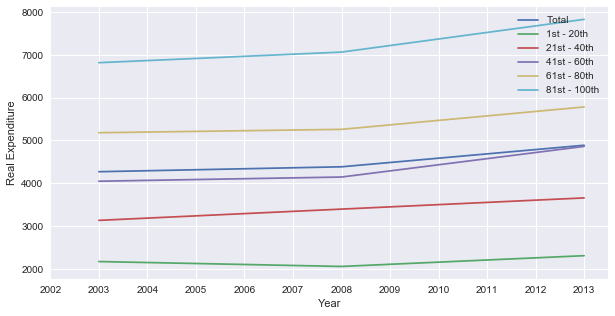Plot above shows real average expenditure per income deciles. Based on initial observation, spending trend increases across all groups.
Since it was only recorded once every 5 years, we cannot get a clear trend on expenditure. With basis to the 2008 Global Financial Crisis, we see a slowing down of expenditure leading to 2008 but consumption picked up after 2008.

Another thing to note is that the difference in expenditure across groups may be due to income restrictions, which may affect the price tier of products that they purchase or the volume of products they purchase. Hence income and expenditure have to be compared together to better analyse the relationship.

As seen in income and expenditure dataframe, the columns do not match. Hence we have to convert the income dataframe to match expenditure.

In :
#Creating another df with the same year range as expenditure
inc = real_inc.copy()

#Since expenditure data is only available between 2002 to 2013 we should filter the income data to match the date
inc = inc[(inc.Year > 2001)&(inc.Year< 2014)]

Out:
Year Total 1st - 10th 11th - 20th 21st - 30th 31st - 40th 41st - 50th 51st - 60th 61st - 70th 71st - 80th 81st - 90th 91st - 100th
2 2002 5814.10 1227.83 2086.56 2802.34 3533.07 4311.71 5172.96 6204.20 7549.44 9529.78 15723.10
3 2003 5808.60 1175.86 2053.53 2821.22 3521.24 4334.96 5226.25 6195.70 7568.99 9444.62 15743.59
4 2004 5890.88 1183.17 2085.08 2824.04 3560.93 4351.41 5126.58 6149.83 7412.38 9554.18 16661.23
5 2005 6150.22 1198.92 2129.58 2923.01 3760.81 4514.05 5416.39 6594.88 7941.06 9830.43 17193.11
6 2006 6331.36 1191.84 2162.70 2969.88 3757.61 4598.89 5547.13 6614.41 8117.13 10130.73 18223.31
In :
#Create seperate dataframes for plotting
#Averging out two decile groups in income df to match expenditure since it is based on 1-20th, 21-40th...

inc_T = inc[['Year','Total']]
inc_1 = inc[['Year']]
inc_1['1st - 20th'] = (inc['1st - 10th'].add(inc['11th - 20th'])).apply(lambda x: x/2)
inc_2 = inc[['Year']]
inc_2 ['21st - 40th'] = (inc['21st - 30th'].add(inc['31st - 40th'])).apply(lambda x: x/2)
inc_3 = inc[['Year']]
inc_3 ['41st - 60th'] = (inc['41st - 50th'].add(inc['51st - 60th'])).apply(lambda x: x/2)
inc_4 = inc[['Year']]
inc_4 ['61st - 80th'] = (inc['61st - 70th'].add(inc['71st - 80th'])).apply(lambda x: x/2)
inc_5 = inc[['Year']]
inc_5 ['81st - 100th'] = (inc['81st - 90th'].add(inc['91st - 100th'])).apply(lambda x: x/2)

In :
#plotting income vs expenditure
fig = plt.figure(figsize =(15,15))
inc_T.plot(kind ='line', x='Year', y ="Total", ax = ax1)
com_exp.plot(kind ='line', x='index', y ="Total", ax = ax1)

ax1.legend(['Income', 'Expenditure'])
ax1.set_title('Total Average Income VS Total Average Expenditure')

inc_1.plot(kind ='line', x='Year', y ="1st - 20th", ax = ax2)
com_exp.plot(kind ='line', x='index', y ="1st - 20th", ax = ax2)
ax2.legend(['Income', 'Expenditure'])
ax2.set_title('Average Income VS Average Expenditure for 1st - 20th Decile')

inc_2.plot(kind ='line', x='Year', y ="21st - 40th", ax = ax3)
com_exp.plot(kind ='line', x='index', y ="21st - 40th", ax = ax3)
ax3.legend(['Income', 'Expenditure'])
ax3.set_title('Average Income VS Average Expenditure for 21st - 40th Decile')

inc_3.plot(kind ='line', x='Year', y ="41st - 60th", ax = ax4)
com_exp.plot(kind ='line', x='index', y ="41st - 60th", ax = ax4)
ax4.legend(['Income', 'Expenditure'])
ax4.set_title('Average Income VS Average Expenditure for 41st - 60th Decile')

inc_4.plot(kind ='line', x='Year', y ="61st - 80th", ax = ax5)
com_exp.plot(kind ='line', x='index', y ="61st - 80th", ax = ax5)
ax5.legend(['Income', 'Expenditure'])
ax5.set_title('Average Income VS Average Expenditure for 61st - 80th Decile')

inc_5.plot(kind ='line', x='Year', y ="81st - 100th", ax = ax6)
com_exp.plot(kind ='line', x='index', y ="81st - 100th", ax = ax6)
ax6.legend(['Income', 'Expenditure'])
ax6.set_title('Average Income VS Average Expenditure for 81st - 100th Decile')

for ax in fig.axes:
plt.sca(ax)
plt.xticks(np.arange(2002,2014,1), rotation=45)
plt.xlabel('Year')
plt.ylabel('Value')
fig.tight_layout()

plt.show()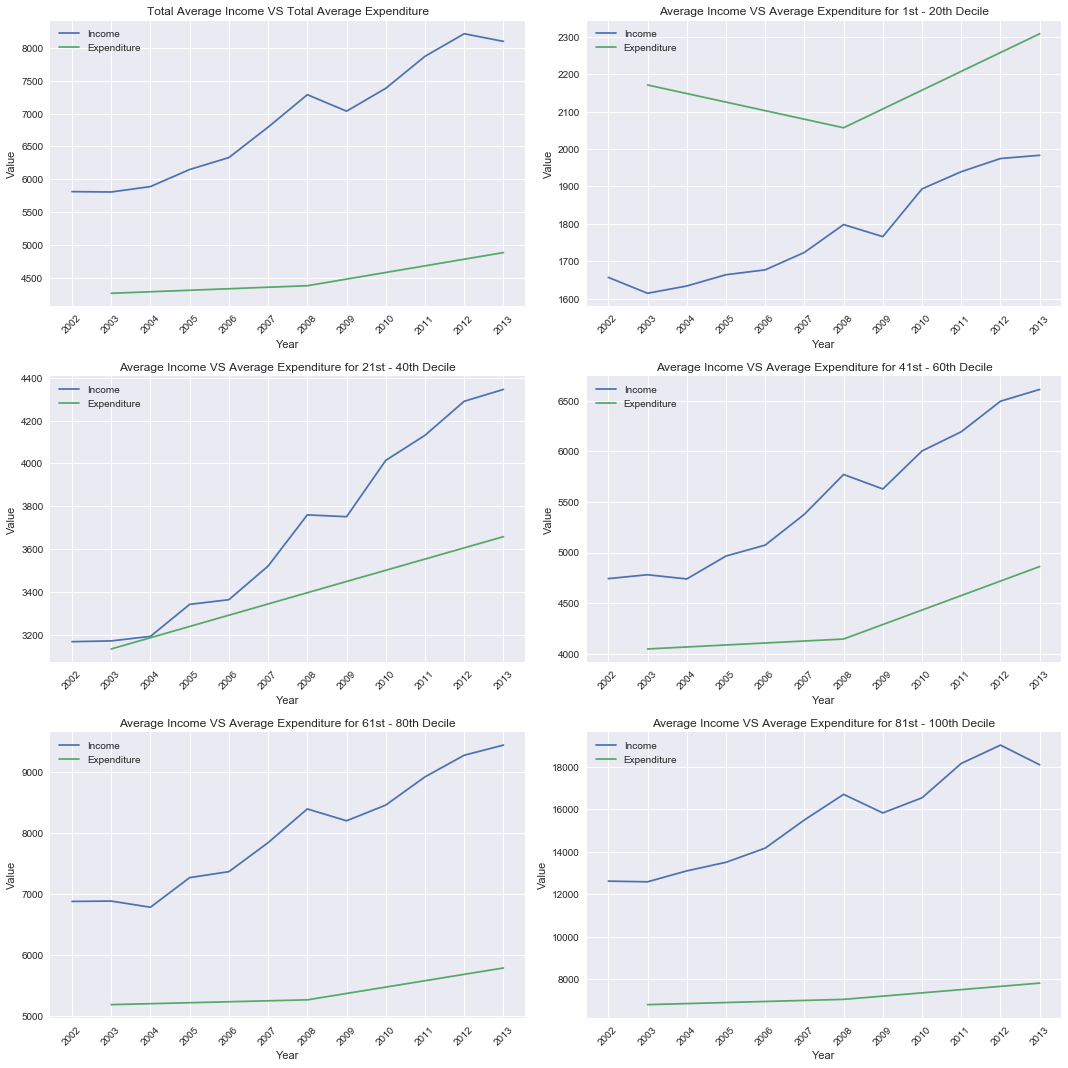Average income and average expenditure were compared in individual subplots for each income decile group. It is noted that expenditure is mostly within a comfortable spending for most income groups.
However, it is observed that only the 0-20th group faced higher expenditure than income by a wide margin, which suggest that there is a lower limit to expenditure which is still unaffordable for this income group. This suggest that this group may be facing urban poverty.

In :
#Based on earlier data, we know 70th percentile and above have income equal or higher than the median
#Hence we will exclude these groups

#Filtering using a new df
inc_poverty = real_inc[['Year','1st - 10th','11th - 20th','21st - 30th','31st - 40th','41st - 50th','51st - 60th','61st - 70th']]

Out:
Year 1st - 10th 11th - 20th 21st - 30th 31st - 40th 41st - 50th 51st - 60th 61st - 70th
0 2000 1383.22 2219.61 2946.20 3624.35 4406.66 5164.13 6138.60
1 2001 1290.39 2177.33 2899.90 3665.17 4422.63 5309.95 6289.49
2 2002 1227.83 2086.56 2802.34 3533.07 4311.71 5172.96 6204.20
3 2003 1175.86 2053.53 2821.22 3521.24 4334.96 5226.25 6195.70
4 2004 1183.17 2085.08 2824.04 3560.93 4351.41 5126.58 6149.83

Singapore does not have an official metric for measuring poverty threshold.Some reports suggest that Singapore uses a 90/10 income measurement which compares the 90th percentile with the 10th percentile. However, that itself shows more of the income ineqaulity and does not indicate at what income level does it fall below poverty threshold. Here we used the metric of two developed countries -UK and Hong Kong

Hong Kong and Singapore are similar in terms of gdp and geographical size. Hong Kong measures the poverty threshold at 50% of median for each income decile, according to South China Sea Post. UK was selected since it has a defined poverty metric of 60% of the median, according to UK charity group (Child Poverty Action Group).

In the earlier income dataset, we do not have median income. Hence we will need to compute using the 41-50th and 51-60th deciles to get an estimation of the median.

In :
#First create new df for median calculations
poverty = real_inc[['Year']]
poverty['median'] = (real_inc['51st - 60th'] + real_inc['41st - 50th'])/2

#Since HK and UK have very close metric, we shall assume threshold at 55% which is the average of the two metrics
poverty['median'] = poverty['median'].apply(lambda x: x*0.55)
poverty

Out:
Year median
0 2000 2631.97
1 2001 2676.46
2 2002 2608.28
3 2003 2629.33
4 2004 2606.45
5 2005 2730.87
6 2006 2790.15
7 2007 2957.54
8 2008 3173.47
9 2009 3094.89
10 2010 3301.33
11 2011 3405.49
12 2012 3571.23
13 2013 3635.31
14 2014 3779.05
15 2015 3924.97
16 2016 3959.73
17 2017 4025.03
In :
# Obtain the mean to compare against the median

mean_poverty = real_inc[['Year']]
mean_poverty['mean'] = real_inc.Total.apply(lambda x: x*0.55)

#Plotting median and mean to observe the difference
fig = plt.figure(figsize=(10,5))
poverty.plot(kind ='line', x = 'Year', marker = 'o', ax = ax)
mean_poverty.plot(kind = 'line',x ='Year', marker = '>', ax=ax)
plt.xticks(np.arange(2000,2018))
plt.show()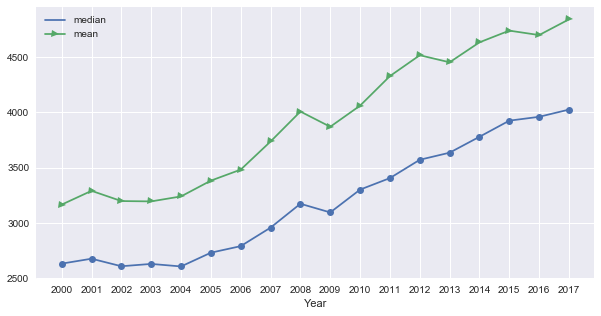Based on the plot, using average instead of median will lead to overestimation of the poverty threshold in Singapore. Hence median will be a better measurement for poverty threshold.

In :
#Plot the graph with poverty against income deciles
#The black line denotes for poverty threshold, any income below the line is considered experiencing poverty

from matplotlib import cm
fig = plt.figure(figsize = (15,10))
ax1 = fig.subplots()
cmap = cm.get_cmap('Spectral')
inc_poverty.plot(kind = 'line',x ='Year' ,ax= ax1, cmap = cmap)
poverty.plot(kind='line', x ='Year', color= 'k',marker ='o', ax= ax1)

ax1.set_xticks(np.arange(2000, 2018))
ax1.set_xlabel('Year')
ax1.set_title('Real Disposable Income VS Poverty Threshold')
ax1.set_ylabel("Real Income")
plt.legend().get_texts().set_text('Poverty Line')
plt.show()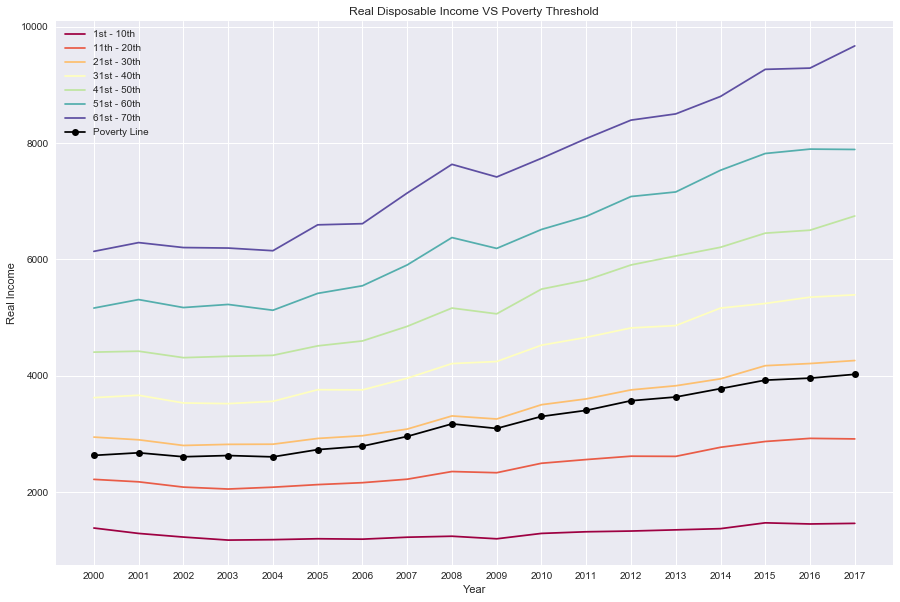Based on international definition, poverty threshold is the minimum income needed to enjoy basic needs in the country. From the plot, with accordance to UK and Hong Kong standard of measure for poverty threshold, it will seem that 20th percentile and below are considered below the poverty threshold. It is also noted that these income groups are still unlikely to improve and cross the threshold, seeing that the line plots are parallel to the poverty line.

Some concerns raised on social media and online platforms indicate fear of a shrinking 'middle-class' and a rising 'upper class' or 'lower class'. It is understandable if we look at the metric so far - 60% of Singaporeans is average and below, 20% can't afford expenditure and below poverty line. However, while the threat of urban poverty is real, the governemnt has to be commented for minimising the widening of the income inequality gap, while ensuring some income increment over the years. Nevertheless, more measures must be taken to help the less fortunate.

Combining all the data observed, the 1-10th and 11-20th decile groups require significant financial attention since these groups are unlikely to cope with Singapore standard of living. With expenditure exceeding income, even with financial support from the government, these income groups cannot escape the cycle of poverty since there is no opportunity to save or invest. This makes it impossible for them to access nor enjoy better amenities or products.

It is also worth noting based on these metrics, poverty threshold is not steepening faster than income increment, which suggest a status quo for now. On another note, while the 21-30th income group remain relatively safe for now, they may face a threat of experiencing urban poverty should income increment rate fall below expenditure increment or poverty threshold increment. Hence the government has to also pay heed to these income groups.

# Related Topics

Sentiment Analysis and Naive Bayes Classification on e-commerce reviews